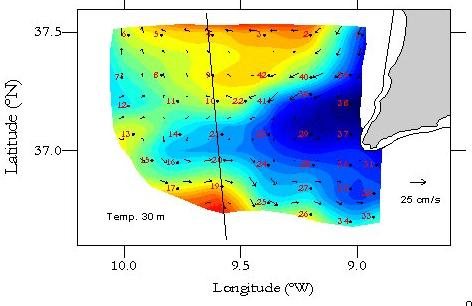You are not Logged in! Log in to check your messages.Check todays hot topics

### Ocean Acoustic Modelling:

The main goal of ocean acoustic modelling is to develop a detailed description of sound propagation in the ocean wave-guide through the calculation of the pressure field between a set of sources and receivers. To this end all propagation models provide an approximate solution of the wave equation, from which the time independent Helmholtz equation can be obtained. The full solution (time dependent) can be obtained by solving the Helmholtz equation at different frequencies and further Fourier synthesis. Besides the incorporation of such static factors as variations of the sound speed along range and depth (and/or variations of the sea surface and bottom) solving the Helmholtz equation requires the development of computational methods to handle such stochastic phenomena as scattering and reverberation.

In general propagation models can be grouped in two categories:

1. Ray models: ray tracers and WKBJ approximations.
2. Wave models: normal modes, fast field and parabolic approximation.

## Ray tracing models

The ray solution of the Helmholtz equation represents a high frequency approximation, which is based on the solution of the Eikonal equation for the phase of the propagating wave, and of the transport equation, which accounts for the waves' amplitude. Ray tracing models are fast to compute and allow to incorporate in a easy way the effects of variations of sound speed and boundary variations over range. The WKBJ approximation can be further used to construct ray-like modes of propagation. Major drawbacks of ray models are related to the breakdown of the solution in the vicinity of focal points and caustics and also due to being unable to handle diffraction.

## Normal modes

Normal mode models expand the solution of the Helmholtz equation on an orthogonal basis of eigenfunctions, which are obtained by solving the so-called normal mode equation. In general each mode represents a pair of opposite oblique waves, with a vertical component which is a standing wave and a horizontal component which propagates away from the source. Those modes can be grouped in two categories:
1. Trapped modes: modes, whose energy does not leave the boundaries of the wave-guide.
2. Evanescent modes: modes, with an amplitude which decreases exponentially along range.
The normal mode expansion of the pressure field is valid as long as sound speed does not depend on range; otherwise, the solution of the Helmholtz equation requires solving the normal mode equation at different ranges and matching of the expansions along range as the wave propagates. That can be done by single mode-to-mode coupling (a solution known as the adiabatic approximation) or by calculation of the coupling coefficients, which is a time consuming procedure.

## Fast field

An alternative to the mode expansion consists in the Fourier decomposition of the acoustic field into an infinite set of horizontal waves, which can be evaluated numerically using the fast Fourier transform. Such numerical solution of the Helmholtz equation lies at the basis of all fast field models, which can be considered as an extension of normal mode models, since the normal mode expansion represents an asymptotic approximation of the fast field integral far from the source. Fast field models provide extremely accurate descriptions of the sound field at low and high frequencies, at short and large ranges from the source, but at the cost of an intensive computational effort.

## Parabolic equation

When the acoustic wave-guide is changing slowly with range the Helmholtz equation can be substituted by the so-called parabolic equation, which differs from the Helmholtz equation by only the first derivative in the r direction. Such substitution has the main numerical advantage that allows an efficient integration of the solution by marching progressively along range. However, as a price to be paid for the substitution it is important to keep in mind that every parabolic equation model provides a solution, which is valid only within a narrow cone against the horizontal.

### Ray Tracing Models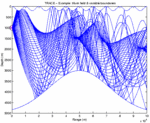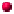BELLHOP: part of the Acoustic Toolbox.TRACEO: ray trace model with several options for the calculation of particle velocity and object scattering.
 Documentation: Manual online PDF Manual C version Downloads: Fortran version C version TraceoTools Python Tools

### Normal Mode Models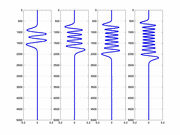KRAKEN: part of the Acoustic Toolbox.SNAP

### Fast Field Models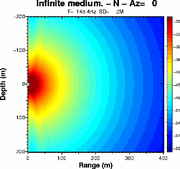OASESSAFARISCOOTER: part of the Acoustic Toolbox.

### Parabolic Equation Models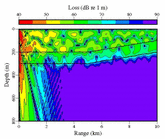RAM

### Direct Time Domain Integration Models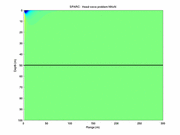SPARC: part of the Acoustic Toolbox.

### Models/InversionSAGA